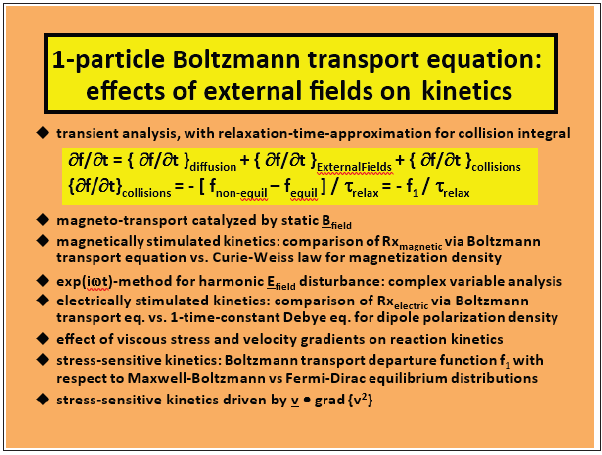• Submissions# Stress- Activated Smart Materials that Respond via Curie Symmetry and the Non- Equilibrium Boltzmann Equation

Laurence A Belfiore*

Polymer Physics & Chemical Reaction Engineering Laboratories Department of Chemical & Biological Engineering, USA

*Corresponding author: Laurence A Belfiore, Polymer Physics & Chemical Reaction Engineering Laboratories, Department of Chemical & Biological Engineering, Colorado State University, and the Aspen Center for Theoretical Physics Fort Collins, Colorado, USA

Submission: October 13, 2020;Published: November 17, 2020

DOI: 10.31031/PSPRJ.2020.01.000510ISSN: 2770-6613
Volume1 Issue2

#### Opinion

Overview

Macromolecules that respond to external stimuli represent a unique class of smart materials. In general, sensitivity to stress in biological macromolecules is described via complex mechano-transduction pathways. Theoretical foundations of stress- kinetic scalar cross-phenomena are evident in the transport-phenomena-based rate of entropy generation and the corresponding linear laws proposed by Onsager, with assistance from Curie’s symmetry theorem. According to Pierre Curie, physical phenomena occur as a consequence of symmetry-breaking, such that systems experience a reduction in symmetry. This concept is supported by Landau free energy functionals for ferromagnets in the vicinity of the Curie temperature (i.e., critical point). The breaking of symmetry has acquired special significance in physics, with respect to the laws of nature. The concept of stress-sensitive chemical transformations in smart materials yields unique rate laws via solution of Boltzmann’s non-equilibrium transport equation that employs a “relaxation-time approximation” to quantify binary collisions between reactive molecules.

Invariance principles

Smart materials that undergo physical or chemical modifications in response to viscous stress are justified theoretically via Curie’s selection theorem in the thermodynamics of irreversible processes. This is an example of stress-kinetic scalar cross phenomena, where transformation rates are coupled to a scalar representation of the (i) velocity gradient tensor, (ii) stress tensor, (iii) rate-of-strain tensor, or (iv) possibly the strain tensor in linear viscoelasticity. Curie’s principles of (i) geometric invariance, otherwise known as reference-frame indifference, and (ii) property invariance with respect to general orthogonal coordinate rotations, have been employed to construct permissible laws of mechanics. In general, the universal symmetry properties of matter with respect to space and time, together with the Lagrangian equations of motion, yield conservation laws in classical and quantum physics according to Emmy Noether’s theorem, where some physical property of the system is a constant of the motion along a trajectory. Mathematical physicist Jules Henri Poincaré stressed the importance of geometric invariance principles for the laws of physics under various coordinate transformations, and he applied these principles to obtain perfect invariance of Maxwell’s equations in electromagnetism.

Boltzmann’s transport equation

According to the molecular fluxes for dissipative processes in transport phenomena, stress-activated processes in macromolecular physics are formulated via appropriate integral averaging with respect to Boltzmann’s non-equilibrium distribution function for molecular velocity. Perturbations from equilibrium are expanded linearly via all macroscopic driving force gradients in the rate of entropy generation. Time-reversal symmetry is broken for stress-activated processes. In other words, the flux expressions are not insensitive to a replacement of time t by –t due to 2nd law irreversibilities and Prigogine’s “arrow of time”, which is a fact of nature that requires explanation. If time-reversal symmetry is obeyed, then particle trajectories viewed forward and backward in time are indistinguishable. It is necessary to distinguish the laws of thermodynamics from the probabilistic nature of entropy which is based on “hidden information” or “memory loss” associated with the decay of correlations among interacting molecules that influence accurate predictions of time evolution (i.e., what happens next). The connection between entropy and hidden information is based on the fact that entropy is contained in a collection of degrees of freedom that is too small to visualize and too numerous to organize. In 1872, Boltzmann invoked the time-asymmetric assumption of Stosszahlansatz, such that the probability of two-particle collisions is expressed as a product of two single-particle distribution functions. The relaxation-time approximation greatly simplifies complex collision terms in Boltzmann’s 1-particle transport equation such that non-equilibrium f1 perturbations can be evaluated analytically in several examples where external fields induce measurable cause-effect relations. (Figure 1), below, provides an overview of Boltzmann transport when chemical transformations in smart materials are catalyzed by magnetic fields, time-varying electric fields, and mechanical stress.

Figure 1: An overview of Boltzmann transport when chemical transformations in smart materials.Stress-sensitive chemical transformations

We at Crimson Publishing are a group of people with a combined passion for science and research, who wants to bring to the world a unified platform where all scientific know-how is available read more...